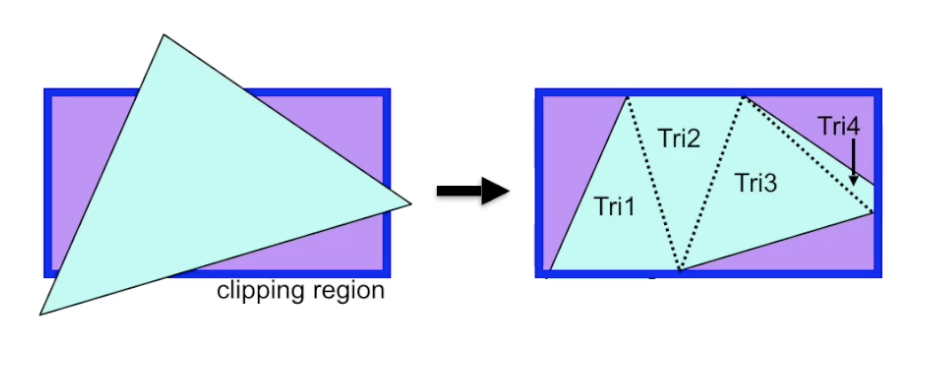# 计算机图形学导论：投影

## Projection

Posted by R1NG on March 6, 2022 Viewed Times

# 投影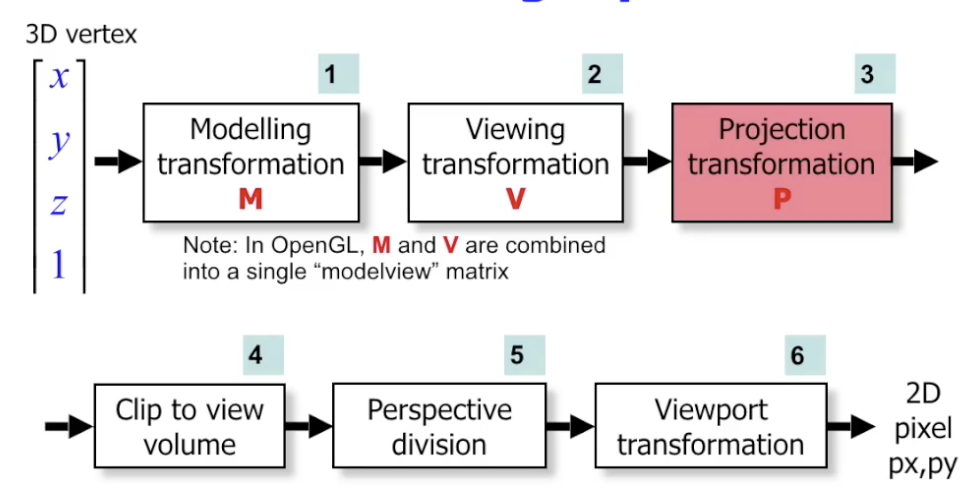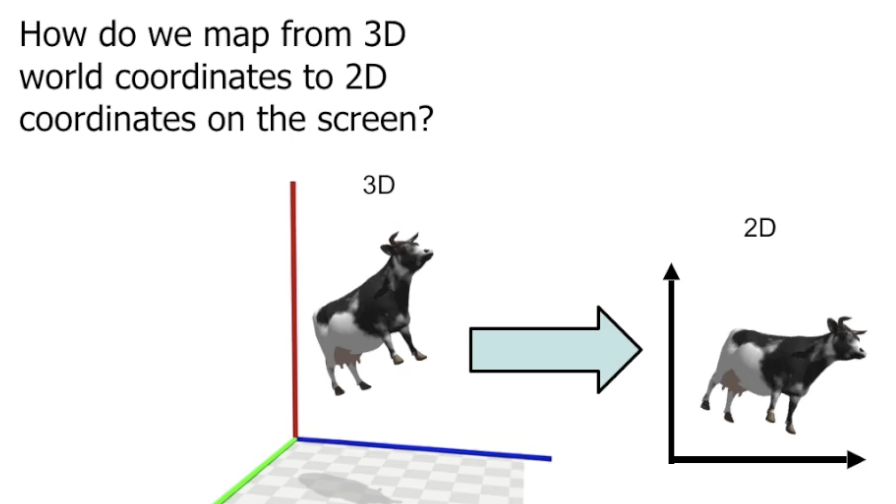## 平行投影 (Parallel Projection)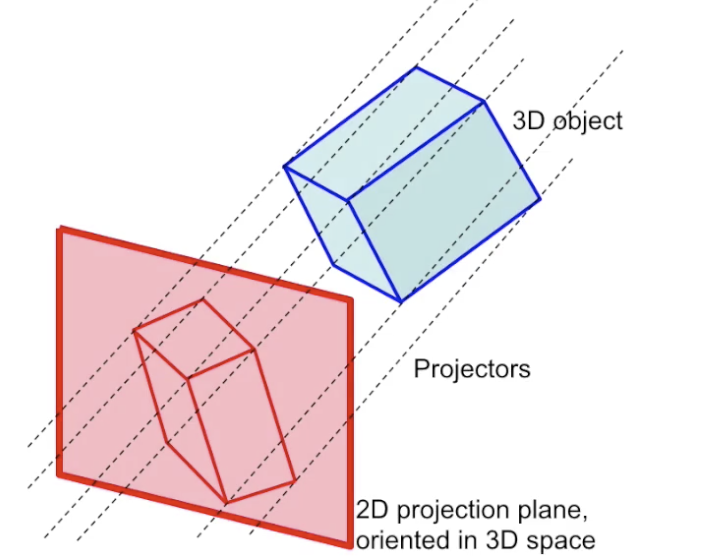### 正交投影 (Orthographic Projection)

#### 三视图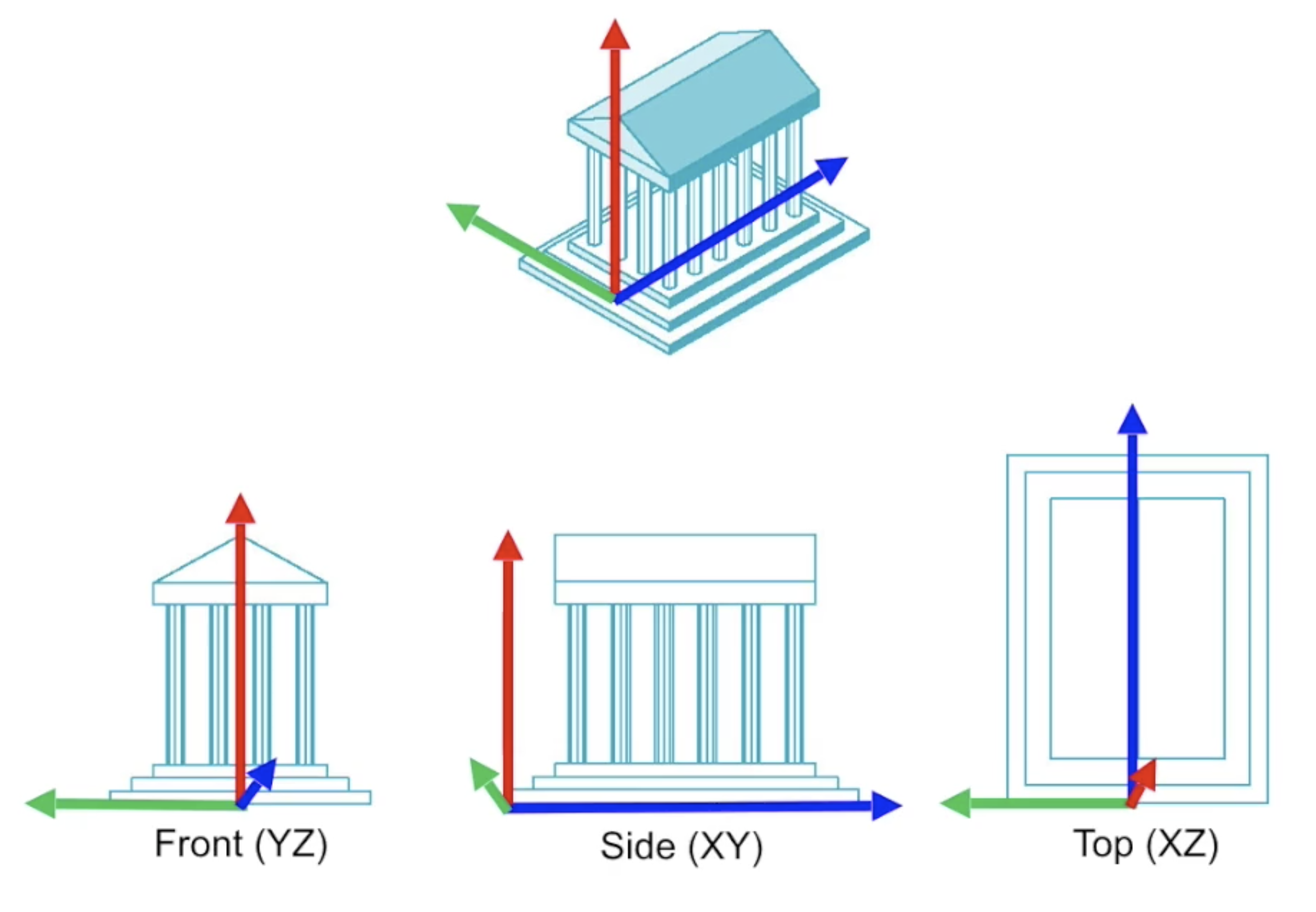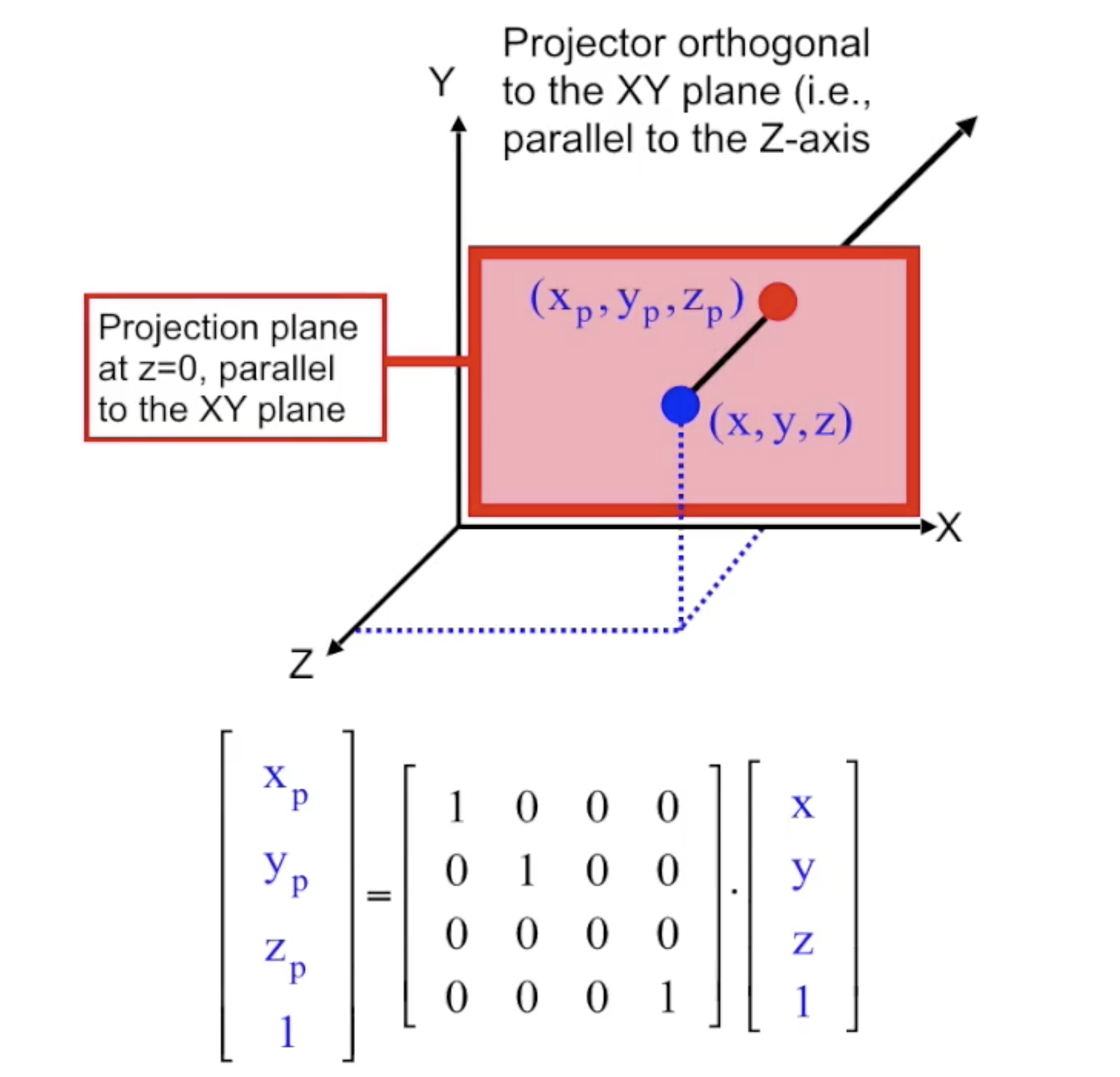#### 斜轴侧投影 (Axonometric Projection)

1. 斜等轴投影 (Isometric Projection):

三个轴向伸缩系数相等, 也就是 $x=y=z$.

2. 斜二轴投影 (Dimetric Projection):

只有两个轴向伸缩系数相等, 也就是 $x=y \neq z$, 或 $x=z \neq y$, 或 $x \neq y=z$.

3. 斜三轴投影 (Trimetric Projection):\

三个轴向伸缩系数均不相同, 即 $x \neq y \neq z$.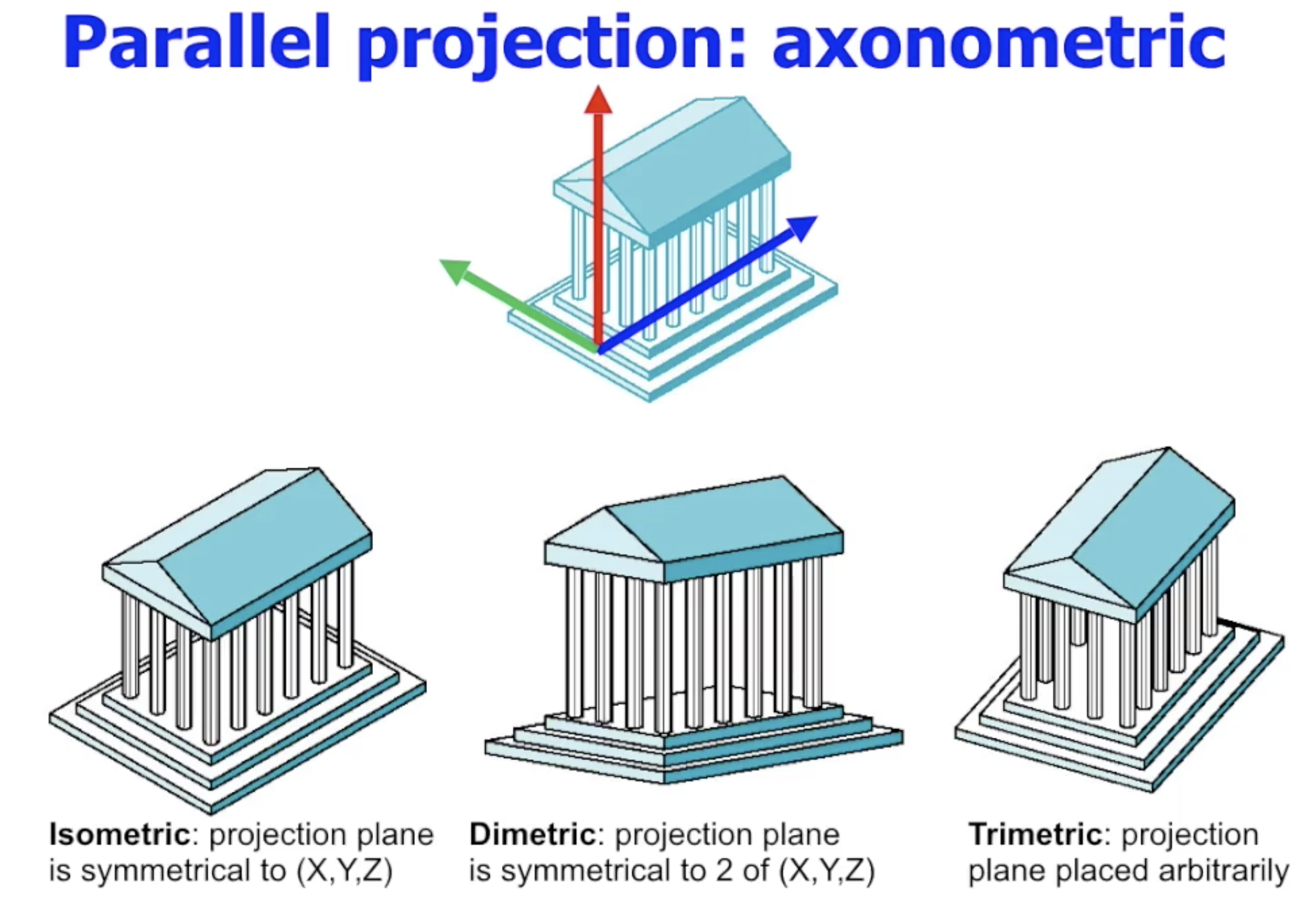#### 倾斜投影 (Oblique Projection)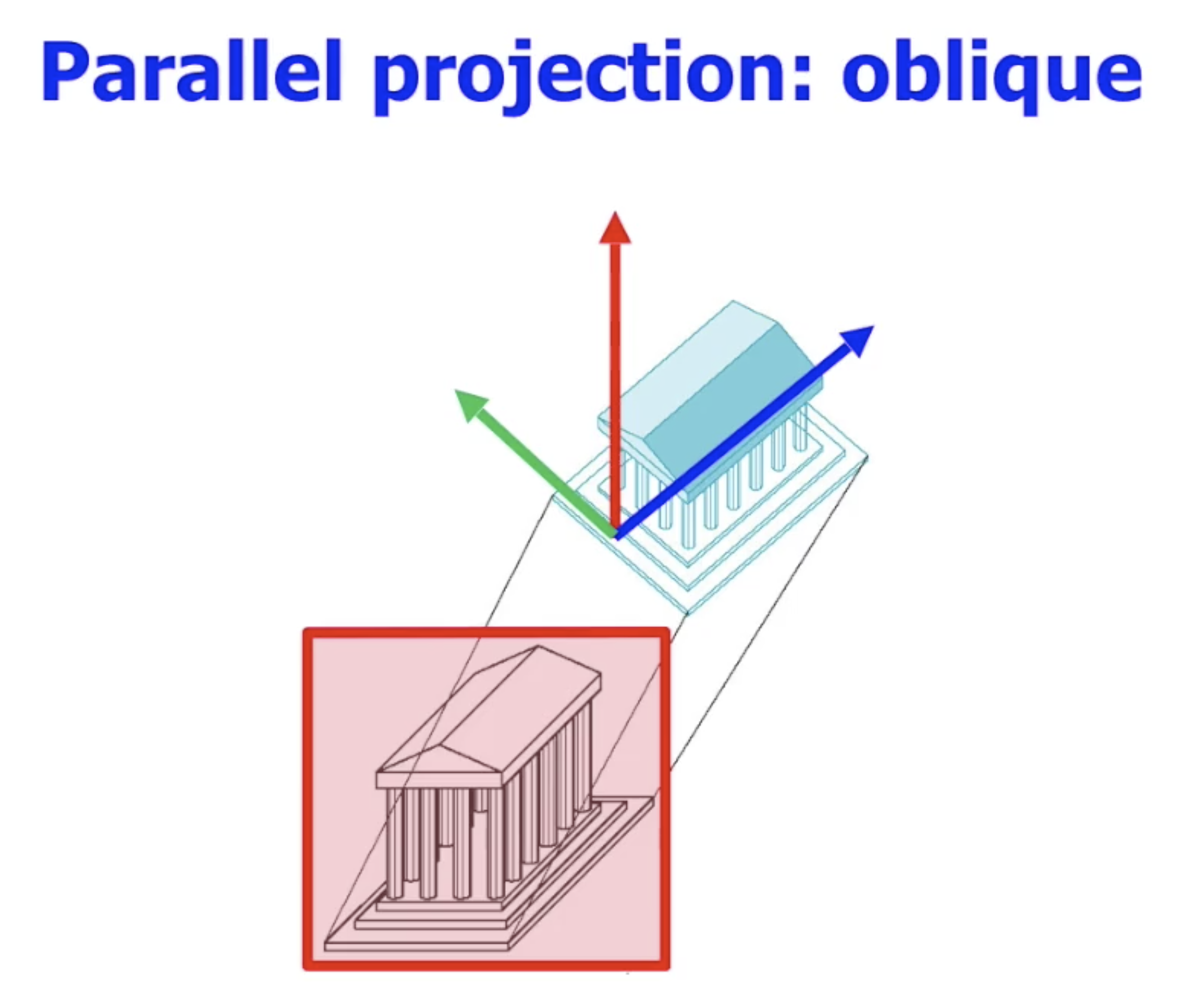## 透视投影 (Perspective Projection)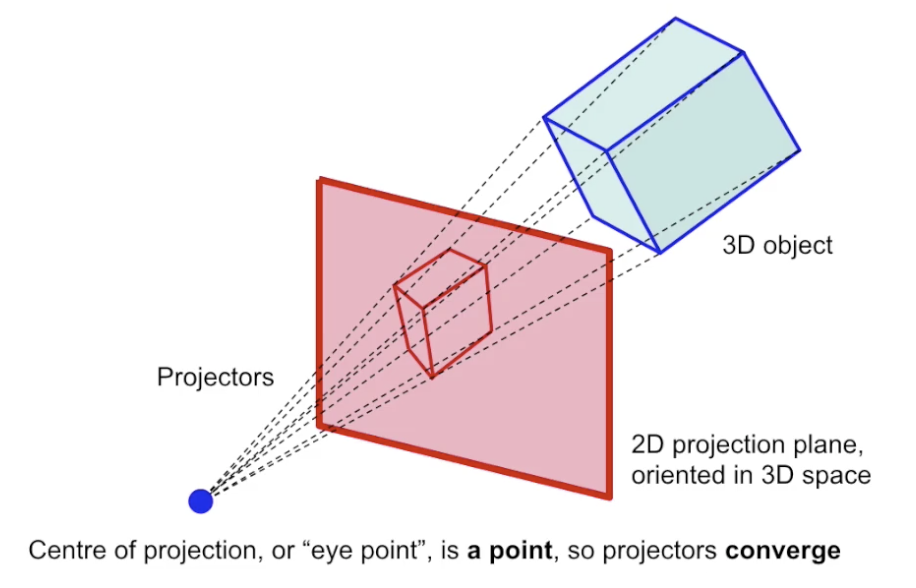1. 1-Point Perspective: 图中平行线如果汇聚, 只会汇聚于一个无穷远点.
2. 2-Point Perspective: 图中平行线如果汇聚, 则可能汇聚于两个无穷远点.
3. 3-Point Perspective: 图中平行线如果汇聚, 则可能会汇聚于三个无穷远点.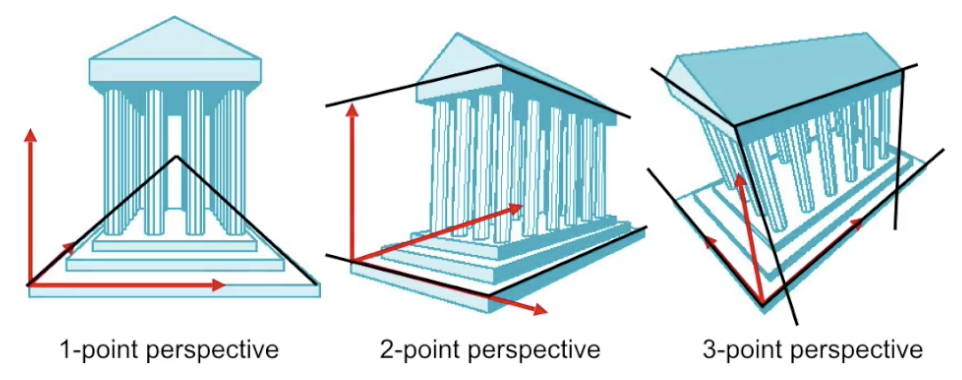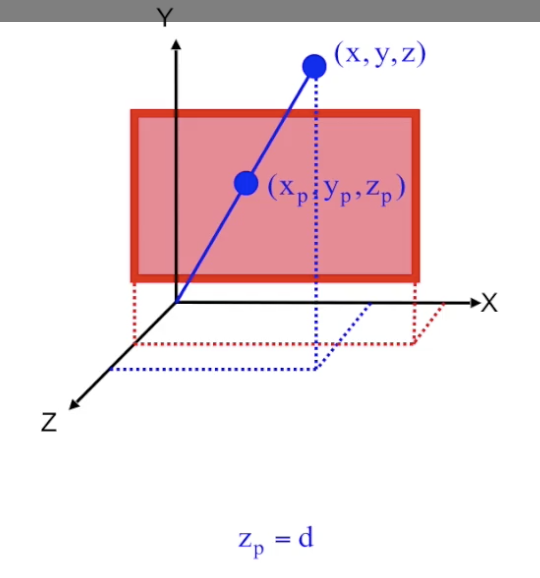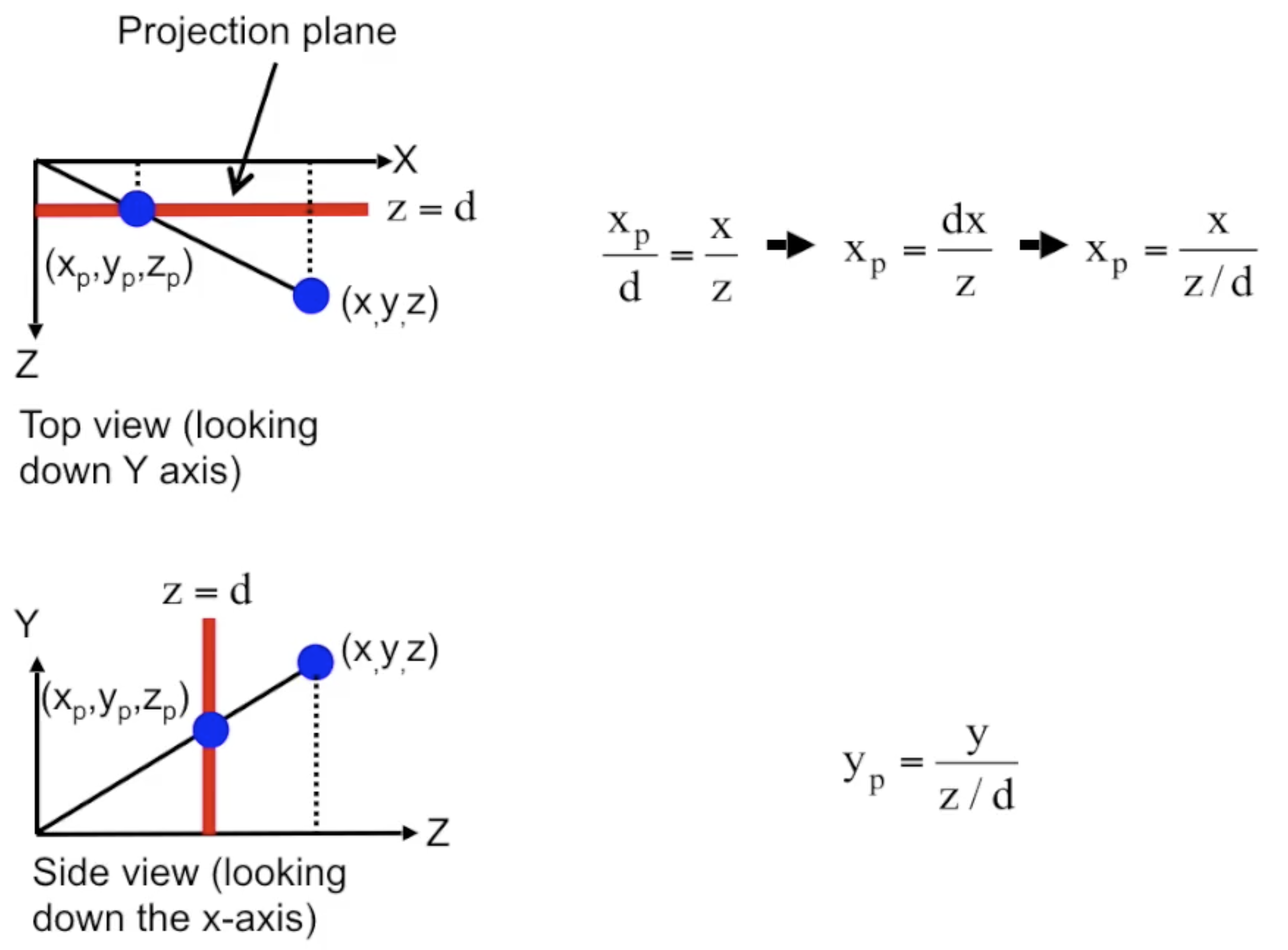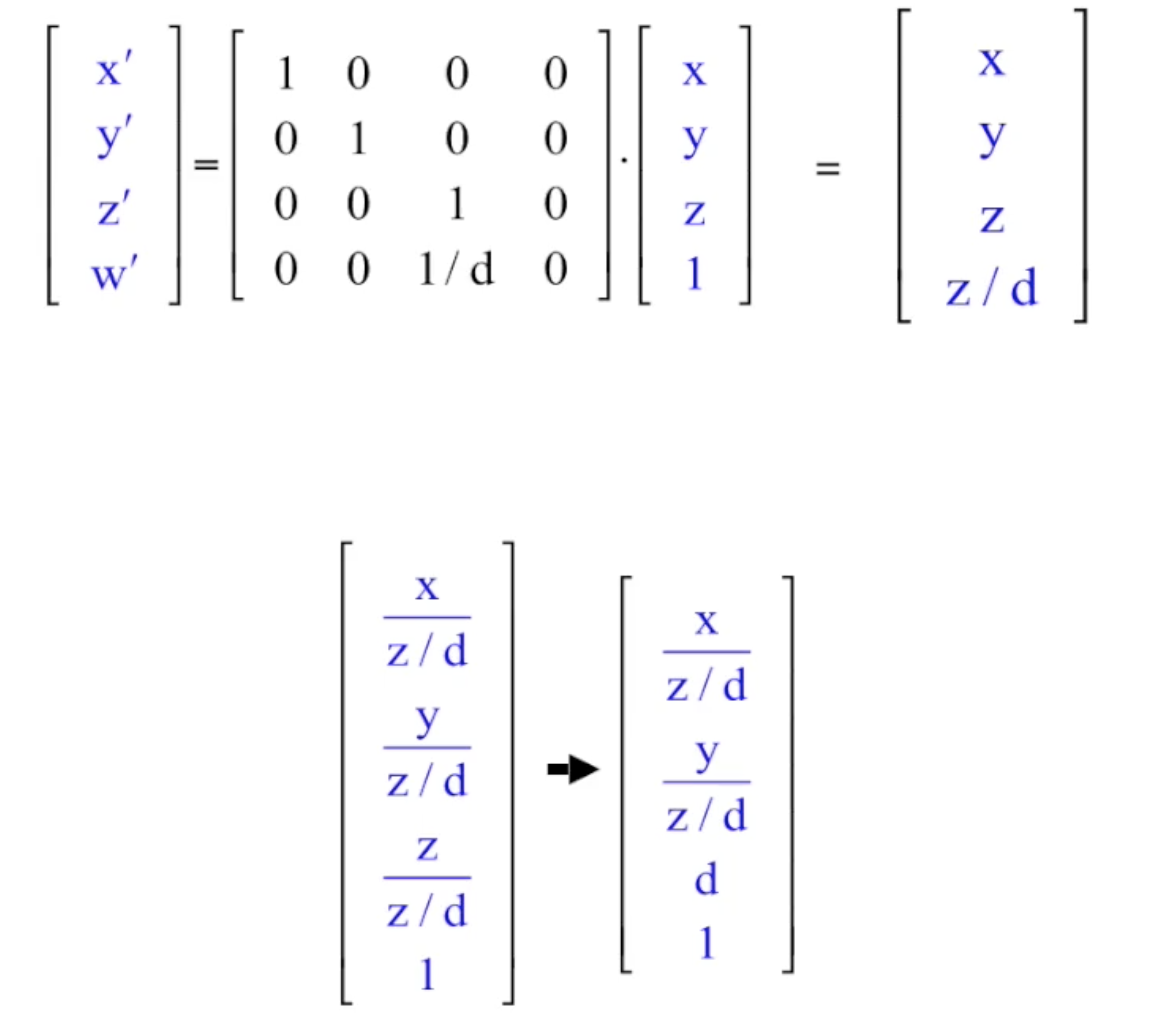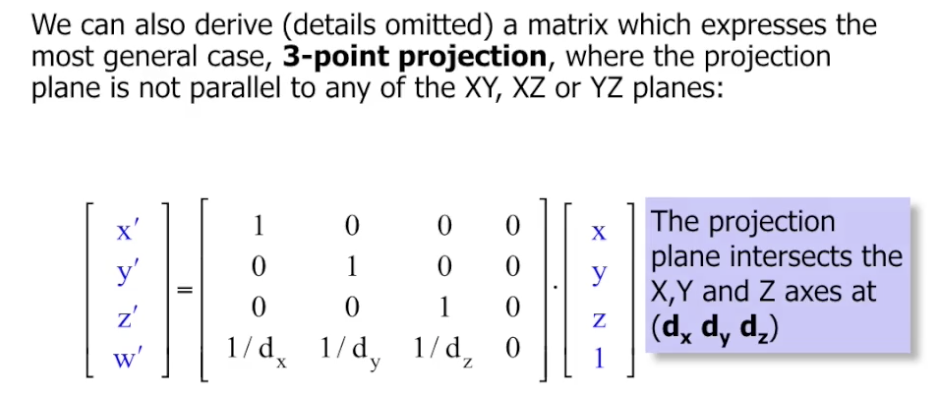## 投影视场 (Field of View)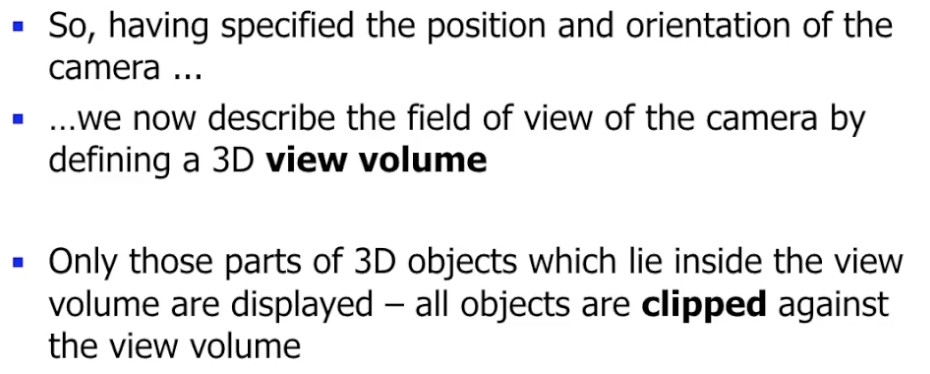### 视景体 (View Volume)

#### 平行投影的视景体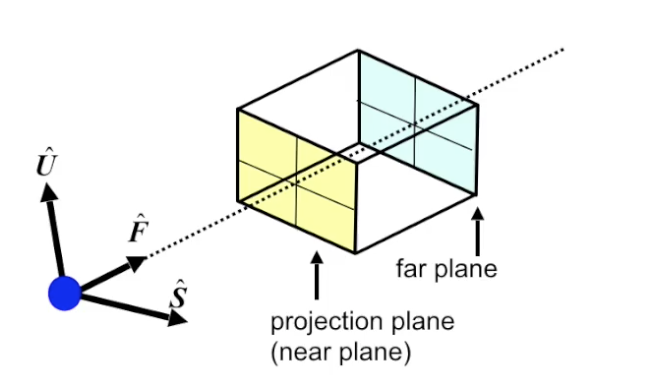#### 正交投影的视景体

ThreeJS 提供了 正交投影相机, 它定义的正交投影视景体就是 由 $6$ 个面组成的长方体, 其中最近面也就是实际的投影面.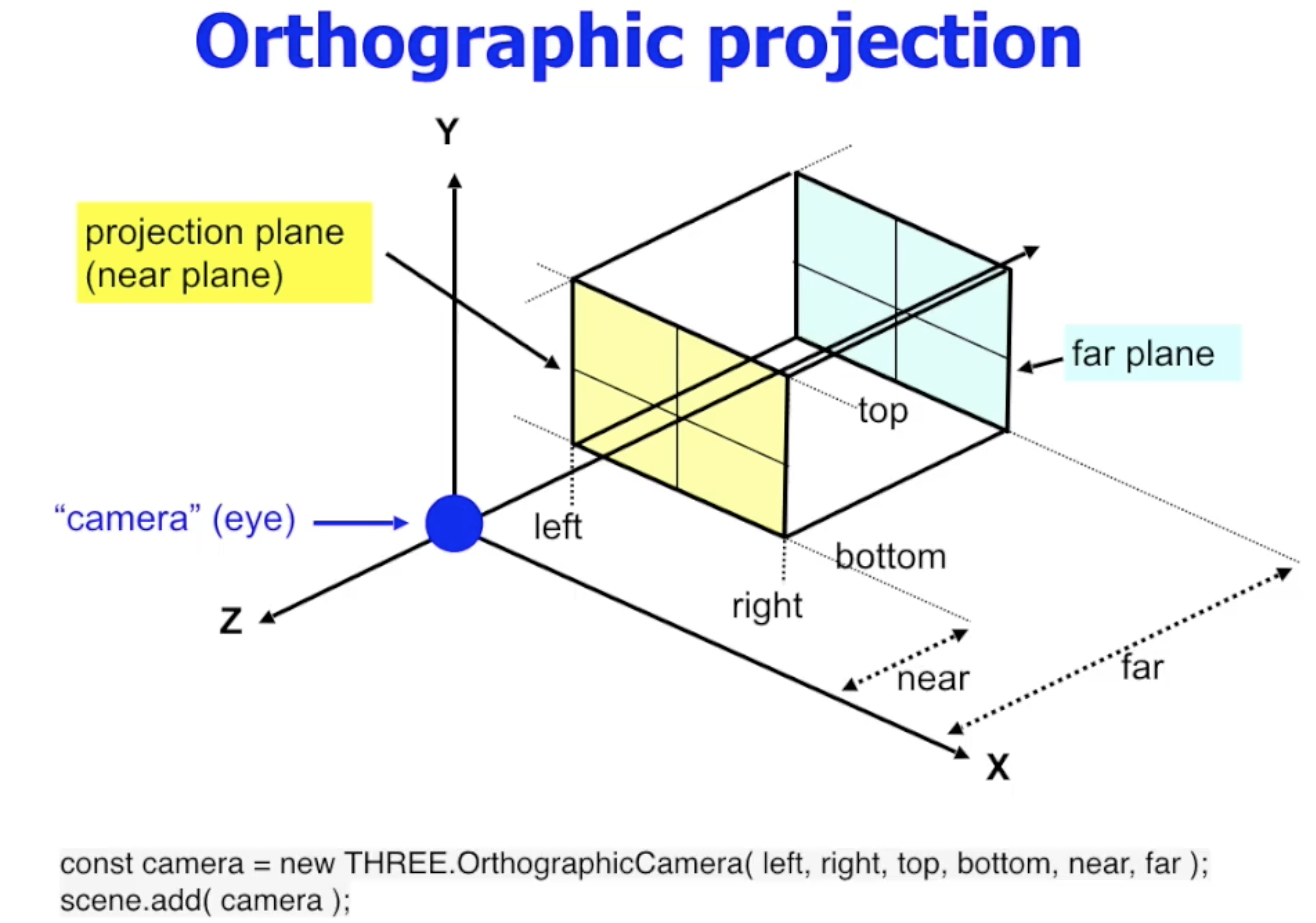#### 透视投影的视景体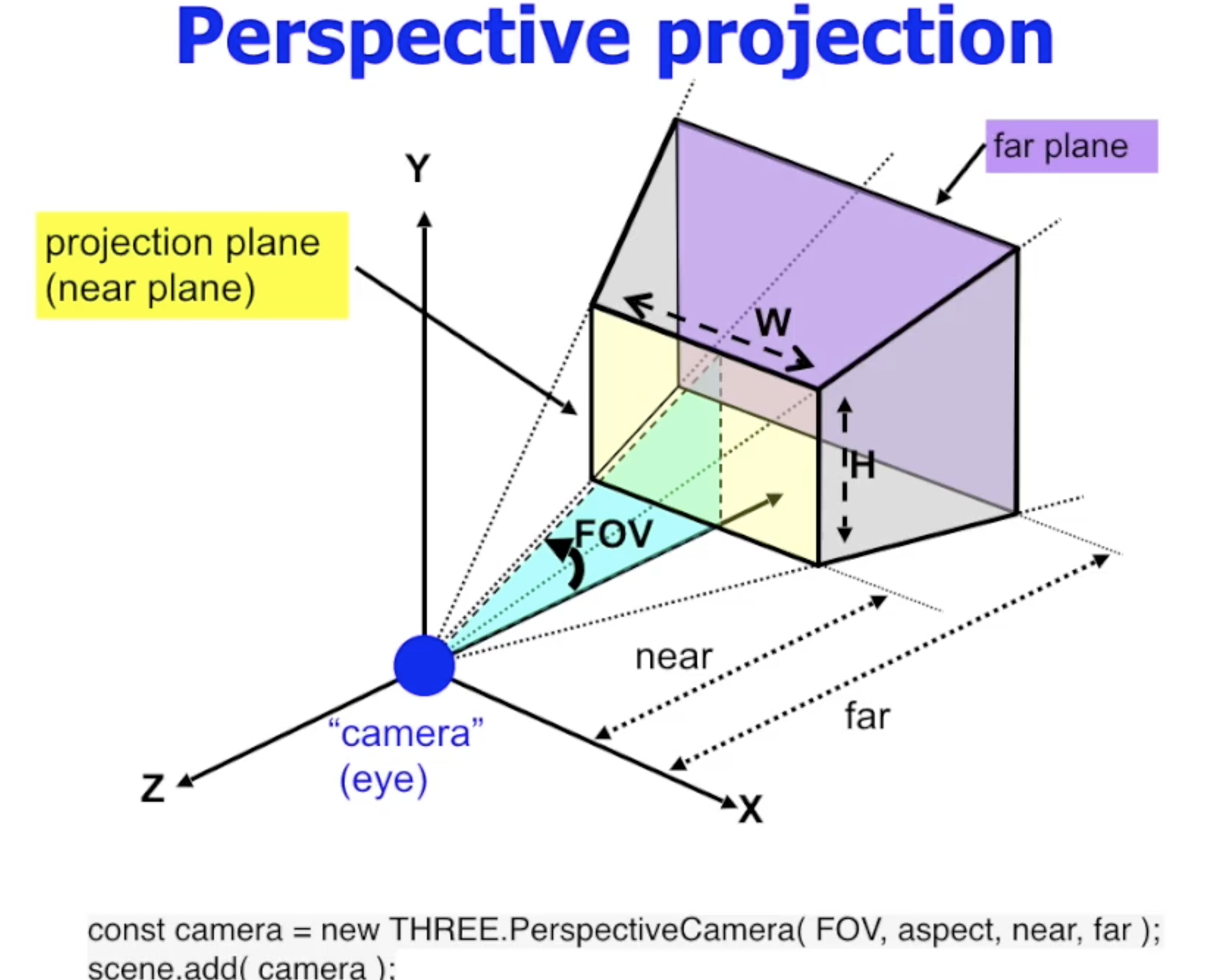#### 投影规范化: 避免透视变换中的信息损失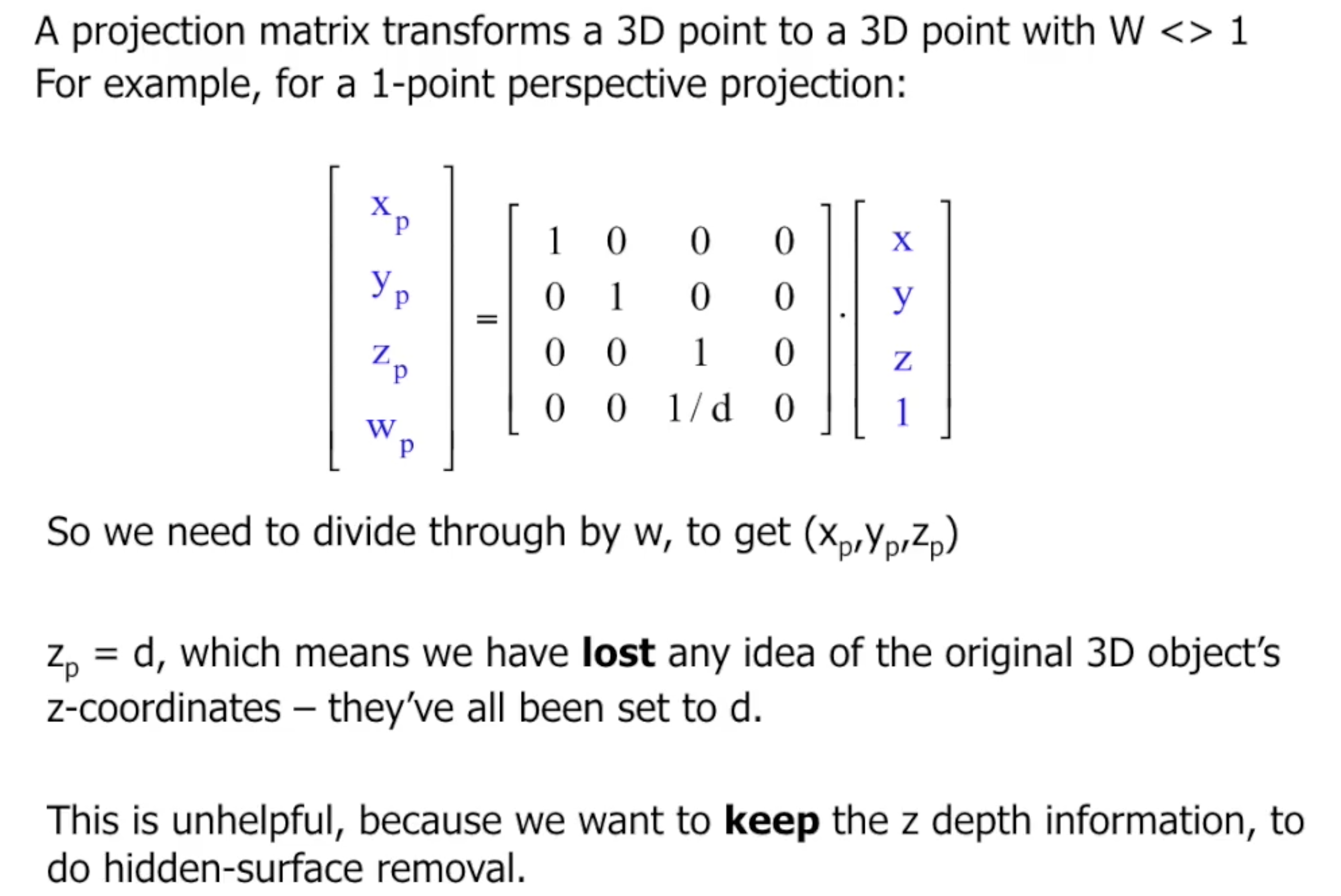$z$ 轴上的信息损失是不可接受的, 因为我们需要它来作为 $z-buffer$ 的数据源, 用来执行 隐藏面移除.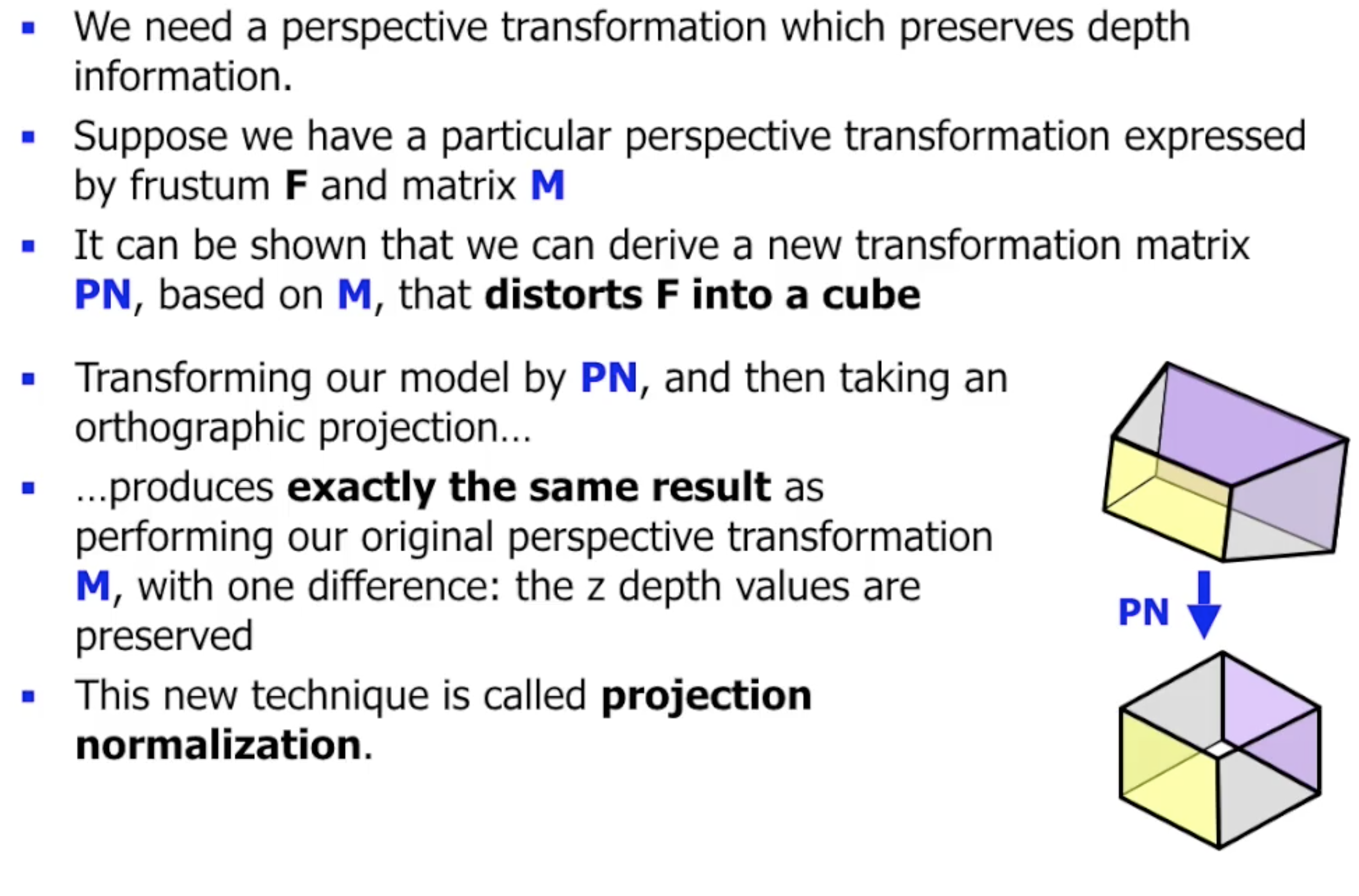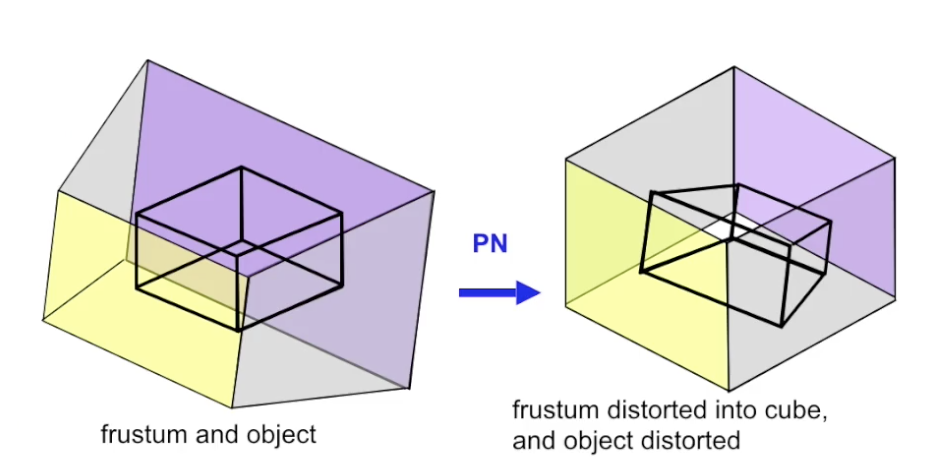### 裁切